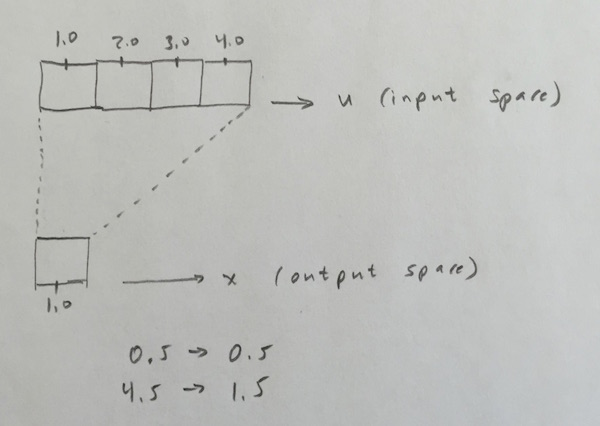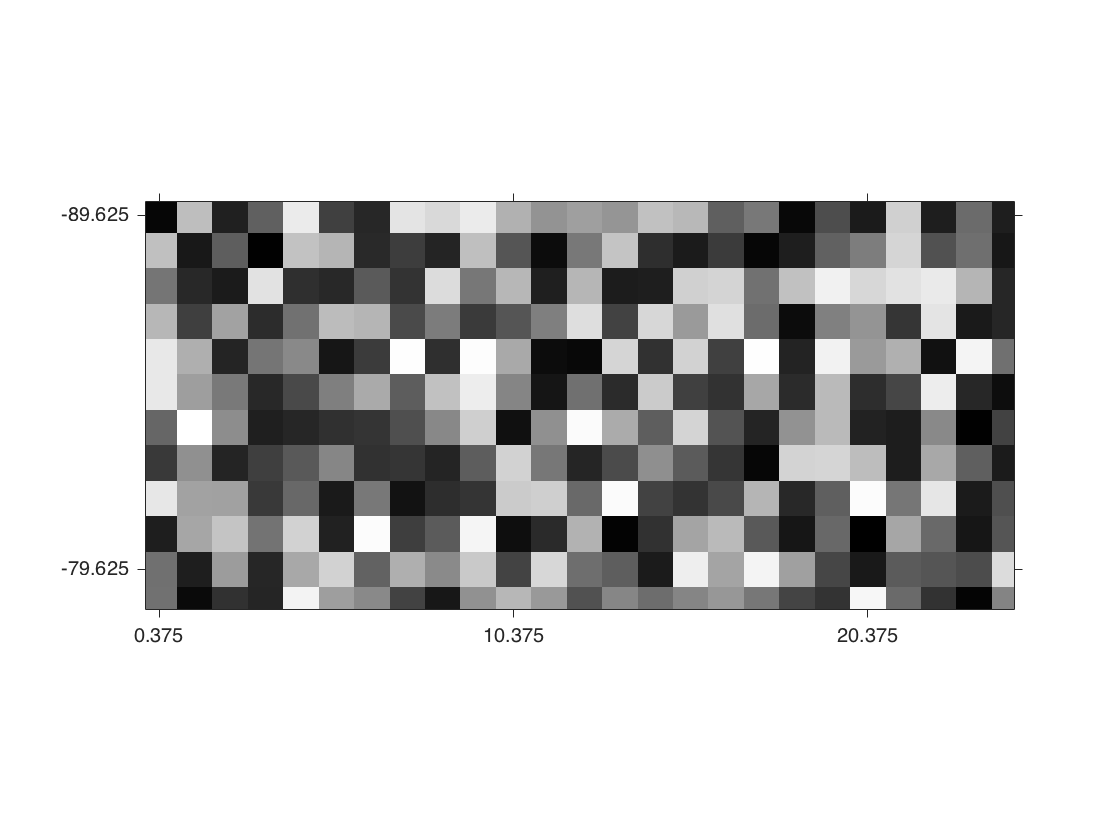# Image resizing geometry

This question landed in my inbox recently:

I have a 721x1441 matrix, T, of temperature values. The x-axis goes from 0 to 360 degrees in steps of 0.25 degrees. The y-axis goes from -90 to 90 degrees, also in steps of 0.25 degrees.

If I call imresize(T,0.25) to shrink the image by a factor of 4, what is the geometry of the result in terms of degrees? Is it 0:360 along the x-axis and -90:90 along the y-axis?

This is a really good question that I would be worth exploring in a blog post. I've personally wrestled enough with image resizing over years to know that there's more than one way to precisely interpret the phrase "shrink by a factor of 4." So let me show you exactly what's happening with imresize.

Suppose you have an 8-by-8 image, and you want to shrink it by a factor of 4. I think that most people would expect the output image to be 2-by-2. And that's what imresize produces. The diagram below illustrates the mapping between a region in the input image and the corresponding region in the output image. The 4-pixel by 4-pixel outlined square region on the left geometrically maps exactly to the 1-pixel by 1-pixel square region on the right.Let's get more specific about the coordinate mapping by looking at just one dimension. I'll use the symbol $u$ to represent the input-space coordinate and $x$ to represent the output-space coordinate. Here's what the geometrical mapping looks like when shrinking by a factor of 4:In the diagram above, we can see that $x=0.5$ maps to $u=0.5$ and $x=1.5$ maps to $u=4.5$.

For a general scale factor $s$, here's the equation:

$$u = \frac{x}{s} + \frac{1}{2}\left(1 - \frac{1}{s}\right)$$

This equation appears directly in the code. Look for this line in imresize.m

u = x/scale + 0.5 * (1 - 1/scale);

The output of imresize(T,0.25) is 181-by-361, so it has 361 columns. So, in ordinary pixel coordinates, we have an x-axis with values 1:361. How do those values correspond to degrees?

To answer that question, first convert from output-space coordinates to input-space coordinates.

x = 1:361;
scale = 0.25;
u = x/scale + 0.5*(1 - 1/scale);


Look at the first few and last few values of u.

u(1:5)

ans =

2.5000    6.5000   10.5000   14.5000   18.5000


u(end-4:end)

ans =

1.0e+03 *

1.4265    1.4305    1.4345    1.4385    1.4425



We know that $u=1$ maps to 0 degrees and $u=1441$ maps to 360 degrees. The equation for that mapping is $d_u = (u-1)/4$. That gives us the degree values for each column of the output image:

d_u = (u-1)/4;

d_u(1:5)

ans =

0.3750    1.3750    2.3750    3.3750    4.3750


d_u(end-4:end)

ans =

356.3750  357.3750  358.3750  359.3750  360.3750



Now let's repeat the process for the vertical coordinates. I'll use $y$ as the vertical output-space symbol and $v$ as the vertical input-space symbol. The mapping from $v$ to degrees is $d_v = v/4 - 90.25$.

y = 1:181;
v = y/scale + 0.5*(1 - 1/scale);
d_v = v/4 - 90.25;

d_v(1:5)

ans =

-89.6250  -88.6250  -87.6250  -86.6250  -85.6250


d_v(end-4:end)

ans =

86.3750   87.3750   88.3750   89.3750   90.3750



So the answer to the original question is: _Each pixel is one degree wide, and the upper left pixel is centered at (0.375 degrees, -89.625 degrees).

You can use the 'XData' and 'YData' parameters when you call imshow to incorporate this information. I'll illustrate with a 181-by-361 image of random values.

I = rand(181,361);
imshow(I,'XData',d_u([1 end]),'YData',d_v([1 end]),...
'InitialMagnification','fit')
axis on
ax = gca;
ax.XTick = d_u(1:10:end);
ax.YTick = d_v(1:10:end);
axis([0 24.5 -90 -78.5])I have suggested to the development team that they make this kind of computation easier in the future, possibly by using the spatial referencing objects that have recently been introduced in the Image Processing Toolbox.

Published with MATLAB® R2015b

|